Elliptica Presents Regular Polyhedra
Computer graphics from the 90s!

Back to Polyhedra

A polyhedron is regular if all its faces are equal regular polygons and all its vertices are alike. There are nine such polyhedra: five convex ones known to the Greeks, and four self intersecting ones discovered more recently. In this picture they are presented to us by Elliptica, who personifies the power and beauty of Mathematics. Elliptica herself is described parametrically by quite a few sines, cosines and exponentiations.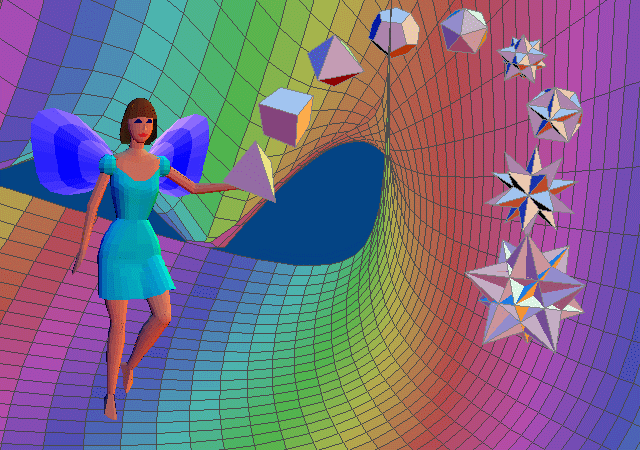Click on the polyhedra below for Elliptica's thoughts on each one.

The polyhedra, starting from Elliptica's hand are:-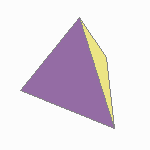Tetrahedron: four triangular faces.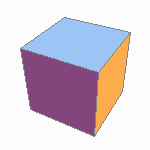Cube (Hexahedron): six square faces.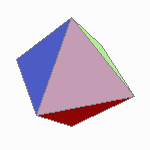Octahedron: eight triangular faces.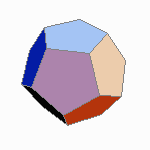Dodecahedron: twelve pentagonal faces.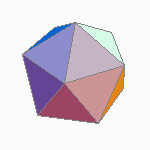Icosahedron: twenty triangular faces.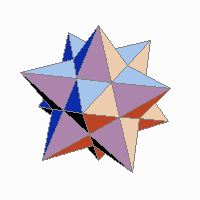Small Stellated Dodecahedron: twelve pentagrammic faces.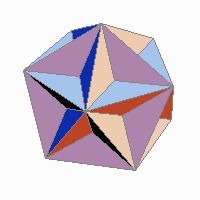Great Dodecahedron: twelve pentagonal faces.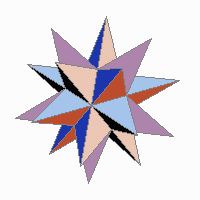Great Stellated Dodecahedron: twelve pentagrammic faces.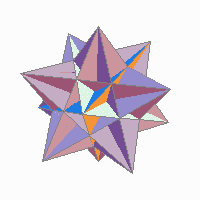Great Icosahedron: twenty triangular faces.

The polyhedra and Elliptica herself have all been rendered using Mathematica.

Back to Polyhedra